Updating search results...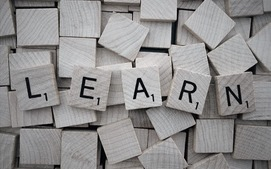# 1 Cluster 1 Lessons

14 affiliated resources

# Search Resources

View
Selected filters: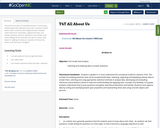Conditional Remix & Share Permitted
CC BY-NC-SA
Rating
0.0 stars

This resource is part of Tools4NCTeachers.

In this lesson, students generate "getting to know you" questions, which lead to both numerical and categorical data. Students collect data from classmates, organize the data, and answer questions about it. Questions may include: How many siblings do you have? Do you like ice cream.

Have ideas for "getting to know you" questions? Remix this lesson, and share your thoughts!

Subject:
Mathematics
Material Type:
Activity/Lab
Lesson
Lesson Plan
11/06/2019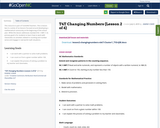Conditional Remix & Share Permitted
CC BY-NC-SA
Rating
0.0 stars

This resource is part of Tools4NCTeachers.

This is lesson two in a series of six lessons focused around developing a mathematical community at the beginning of the school year. While this lesson addresses standard NC.1.NBT.7, its primary goal is for students to learn how to work with classmates on activities related to counting and number sense and engage in real-world math situations.

Subject:
Mathematics
Material Type:
Lesson
Lesson Plan
11/06/2019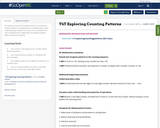Conditional Remix & Share Permitted
CC BY-NC-SA
Rating
0.0 stars

This resource is part of Tools4NCTeachers.

In this lesson, students explore counting patterns to develop place value understanding. Students use tools such as number lines, and a hundred chart. Additionally, the explore patterns within real world situations.

Subject:
Mathematics
Material Type:
Activity/Lab
Lesson
Lesson Plan
11/06/2019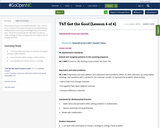Conditional Remix & Share Permitted
CC BY-NC-SA
Rating
0.0 stars

This resource is part of Tools4NCTeachers.

This is lesson six in a series of six lessons focused around developing a mathematical community at the beginning of the school year. The goal is to promote the idea that it is okay to make mistakes in math as long as we try to figure out the mistake and fix it.

Subject:
Mathematics
Material Type:
Lesson
Lesson Plan
11/06/2019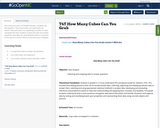Conditional Remix & Share Permitted
CC BY-NC-SA
Rating
0.0 stars

This resource is part of Tools4NCTeachers.

Students ask the question, "How many cubes can I grab with one hand?" They each take a turn grabbing a handful of cubes, and graph the data. Students analyze the data and answer questions

Have ideas for extending this lesson, or pictures of this lesson in action? Remix it, and share your thoughts!

Subject:
Mathematics
Material Type:
Activity/Lab
Lesson
Lesson Plan
11/06/2019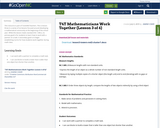Conditional Remix & Share Permitted
CC BY-NC-SA
Rating
0.0 stars

This resource is part of Tools4NCTeachers.

This is lesson three in a series of six lessons focused around developing a mathematical community at the beginning of the school year. While this lesson meets standard NC.1.MD.2, its primary goal is for students to learn how to work with a partner on a task. A secondary goal is to begin establishing norms for how students work together during math class.

Subject:
Mathematics
Material Type:
Lesson
Lesson Plan
11/06/2019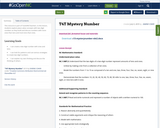Conditional Remix & Share Permitted
CC BY-NC-SA
Rating
0.0 stars

This resource is part of Tools4NCTeachers.

In this lesson, students explore place value concepts with two-digit numbers as they build and discuss numbers with more ones than tens and more tens than ones.

Subject:
Mathematics
Material Type:
Activity/Lab
Lesson
Lesson Plan
11/06/2019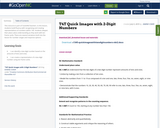Conditional Remix & Share Permitted
CC BY-NC-SA
Rating
0.0 stars

This resource is part of Tools4NCTeachers.

In this lesson, students build fluency with recognizing and creating representations of numbers within 100. Students deepen their place value understanding as they work with ten frame cards. There are several variations built into the lesson for number ranges and response options.

Subject:
Mathematics
Material Type:
Activity/Lab
Lesson
Lesson Plan
11/06/2019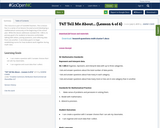Conditional Remix & Share Permitted
CC BY-NC-SA
Rating
0.0 stars

This resource is part of Tools4NCTeachers.

This is lesson four in a series of six lessons focused around developing a mathematical community at the beginning of the school year. While this lesson addresses standard NC.1.MD.4, its primary goal is for students to become comfortable talking with others, posing questions, and collecting data from one another. A secondary goal is to begin establishing norms for how students work together during math class.

Subject:
Mathematics
Material Type:
Lesson
Lesson Plan
11/06/2019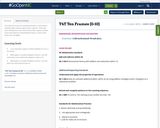Conditional Remix & Share Permitted
CC BY-NC-SA
Rating
0.0 stars

This resource is part of Tools4NCTeachers.

In this lesson, students play a partner game with a spinner. They take turns finding one more and one less than a given number.

If you have ideas for extending this lesson, remix it and share your thoughts!

Subject:
Mathematics
Material Type:
Activity/Lab
Lesson
Lesson Plan
11/06/2019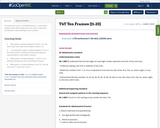Conditional Remix & Share Permitted
CC BY-NC-SA
Rating
0.0 stars

This resource is part of Tools4NCTeachers.

In this lesson, students work with double ten frame mats to represent and develop understanding of teen numbers.

Subject:
Mathematics
Material Type:
Activity/Lab
Lesson
Lesson Plan
11/06/2019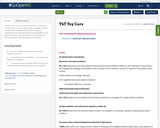Conditional Remix & Share Permitted
CC BY-NC-SA
Rating
0.0 stars

This resource is part of Tools4NCTeachers.

In this lesson, students solve a word problem using a strategy of choice and share the strategy to develop the concept of representing and solving problems involving addition and subtraction within 20. This lesson format may be used with any problem type appropriate for the grade.

Subject:
Mathematics
Material Type:
Lesson
Lesson Plan
11/06/2019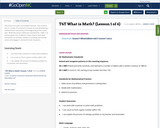Conditional Remix & Share Permitted
CC BY-NC-SA
Rating
0.0 stars

This resource is part of Tools4NCTeachers.

This is lesson one in a series of six lessons focused around developing a mathematical community at the beginning of the school year. While this lesson addresses standard NC.1.NBT.7, its primary goal is for students to learn how to work with classmates on activities related to counting and number sense and engage in real-world math situations.

Subject:
Mathematics
Material Type:
Lesson
Lesson Plan
11/06/2019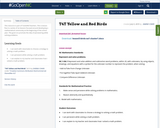Conditional Remix & Share Permitted
CC BY-NC-SA
Rating
0.0 stars

This resource is part of Tools4NCTeachers.

This is lesson five in a series of six lessons focused around developing a mathematical community at the beginning of the school year. The goal is to promote the idea of persevering while solving problems.

Subject:
Mathematics
Material Type:
Lesson
Lesson Plan